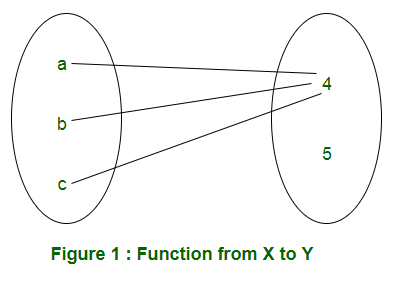# Mathematics | Total number of possible functions

• Difficulty Level : Easy
• Last Updated : 06 Dec, 2021

In this article, we are discussing how to find number of functions from one set to another. For understanding the basics of functions, you can refer this: Classes (Injective, surjective, Bijective) of Functions

Number of functions from one set to another: Let X and Y are two sets having m and n elements respectively. In a function from X to Y, every element of X must be mapped to an element of Y. Therefore, each element of X has ‘n’ elements to be chosen from. Therefore, total number of functions will be n×n×n.. m times = nm
For example: X = {a, b, c} and Y = {4, 5}. A function from X to Y can be represented in Figure 1.Considering all possibilities of mapping elements of X to elements of Y, the set of functions can be represented in Table 1.Examples: Let us discuss gate questions based on this:

• Q1. Let X, Y, Z be sets of sizes x, y and z respectively. Let W = X x Y. Let E be the set of all subsets of W. The number of functions from Z to E is:
(A) z2xy
(B) z x 2xy
(C) z2x + y
(D) 2xyz

Solution: As W = X x Y is given, number of elements in W is xy. As E is the set of all subsets of W, number of elements in E is 2xy. The number of functions from Z (set of z elements) to E (set of 2xy elements) is 2xyz. So the correct option is (D)

• Q2. Let S denote the set of all functions f: {0,1}4 → {0,1}. Denote by N the number of functions from S to the set {0,1}. The value of Log2Log2N is ______.
(A) 12
(B) 13
(C) 15
(D) 16

Solution: As given in the question, S denotes the set of all functions f: {0, 1}4 → {0, 1}. The number of functions from {0,1}4 (16 elements) to {0, 1} (2 elements) are 216. Therefore, S has 216 elements. Also, given, N denotes the number of function from S(216 elements) to {0, 1}(2 elements). Therefore, N has 2216 elements. Calculating required value,

Log2(Log2 (2216)) =Log216 = 16

Therefore, correct option is (D).

Number of onto functions from one set to another – In onto function from X to Y, all the elements of Y must be used. In the example of functions from X = {a, b, c} to Y = {4, 5}, F1 and F2 given in Table 1 are not onto. In F1, element 5 of set Y is unused and element 4 is unused in function F2. So, total numbers of onto functions from X to Y are 6 (F3 to F8).

• If X has m elements and Y has 2 elements, the number of onto functions will be 2m-2.

Explanation: From a set of m elements to a set of 2 elements, the total number of functions is 2m. Out of these functions, 2 functions are not onto (If all elements are mapped to 1st element of Y or all elements are mapped to 2nd element of Y). So, number of onto functions is 2m-2.

• If X has m elements and Y has n elements, the number if onto functions are,Important notes –

• The formula works only if m ≥ n.
• If m < n, the number of onto functions is 0 as it is not possible to use all elements of Y.

Q3. The number of onto functions (surjective functions) from set X = {1, 2, 3, 4} to set Y = {a, b, c} is:
(A) 36
(B) 64
(C) 81
(D) 72

Solution: Using m = 4 and n = 3, the number of onto functions is:
343C1(2)4 + 3C214 = 36.

My Personal Notes arrow_drop_up